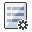The Rocscience International Conference 2021 Proceedings are now available. Read Now

# Tension Crack

If you are performing a Probabilistic analysis with SWedge, and your model includes a Tension Crack, you can define the orientation of the Tension Crack as a Random Variable, as follows:

1. Select Input Dataand select the Tension Crack tab in the Probabilistic Input Data dialog.
2. Select the Tension Crack check box.
3. Select the Orientation Definition Method. There are two methods of defining the variability of the Tension Crack orientation:
• Dip / Dip Direction
• Fisher Distribution
4. See below for information about these options.

5. Enter or import the Tension Crack mean orientation and statistical parameters, as described below.

## Dip / Dip Direction

If the Orientation Definition Method = Dip / Dip Direction, then the Dip and Dip Direction of the Tension Crack can each be assigned a statistical distribution, and are treated as independent random variables (i.e. during the statistical sampling, there is no correlation between the generated values of Dip and Dip Direction).

The Dip / Dip Direction method allows you the flexibility to choose any two distributions for the Dip and Dip Direction. This can be useful if the actual distribution of orientations you are trying to model, is asymmetric (e.g. an elliptical distribution on the stereonet). However, if your orientation distribution is approximately circular, then you should use the Fisher Distribution option.

## Fisher Distribution

If the Orientation Definition Method = Fisher Distribution, then the Tension Crack orientations will be randomly generated according to a Fisher Distribution. A Fisher Distribution assumes a symmetric distribution of 3-dimensional orientations around the mean, and only requires a single parameter (Fisher K value or standard deviation) to define the variability of the distribution. Note: the Fisher K value and the standard deviation of a Fisher distribution are related, so you can use either parameter.